International
Tables for
Crystallography
Volume B
Reciprocal space
Edited by U. Shmueli

International Tables for Crystallography (2006). Vol. B, ch. 5.1, pp. 540-541   | 1 | 2 |

## Section 5.1.3.7. Geometrical interpretation of the solution in the zero-absorption case

A. Authiera*

aLaboratoire de Minéralogie-Cristallographie, Université P. et M. Curie, 4 Place Jussieu, F-75252 Paris CEDEX 05, France
Correspondence e-mail: authier@lmcp.jussieu.fr

| top | pdf |

#### 5.1.3.7.1. Transmission geometry

| top | pdf |

In this case (Fig. 5.1.3.4)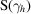is +1 and (5.1.3.10)may be written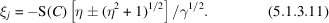Letandbe the intersections of the normal to the crystal surface drawn from the Lorentz point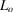with the two branches of the dispersion surface (Fig. 5.1.3.4). From Sections 5.1.3.3and 5.1.3.4, they are the tie points excited for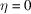and correspond to the middle of the reflection domain. Let us further consider the tangents to the dispersion surface atandand let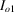,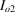and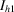,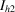be their intersections with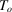and, respectively. It can be shown that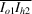and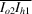intersect the dispersion surface at the tie points excited for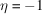and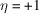, respectively, and that the Pendellösung distance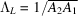, the width of the rocking curve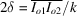and the deviation parameter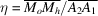, where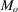and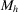are the intersections of the normal to the crystal surface drawn from the extremity of any incident wavevector OM withand, respectively.

#### 5.1.3.7.2. Reflection geometry

| top | pdf |

In this case (Fig. 5.1.3.5)is now −1 and (5.1.3.10)may be written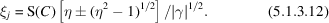Now, letandbe the points of the dispersion surface where the tangent is parallel to the normal to the crystal surface, and further let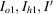and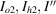be the intersections of these two tangents with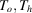and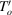, respectively. For an incident wave of wavevector OM where M lies between I′ and I″, the normal to the crystal surface drawn from M has no real intersection with the dispersion surface and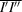defines the total-reflection domain. The tie pointsandcorrespond toand, respectively, the extinction distance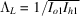, the width of the total-reflection domain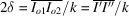and the deviation parameter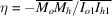, whereandare the intersections withandof the normal to the crystal surface drawn from the extremity of any incident wavevector OM.